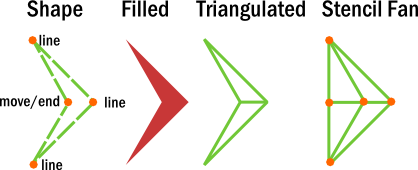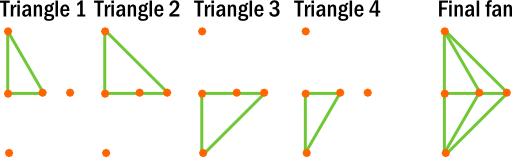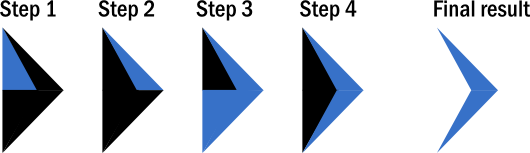# articles

Triangulation can be a computationally expensive task if done in excess, regardless of the method used. In turn, performance in a real time simulation, such as a video game, can be affected in a negative manner by highly dynamic graphics, especially when shapes are created every frame. However, one can make use of the stencil buffer to offset much of the complex triangulation load, outlined below.

## Concave Polygon Triangulation and the Stencil Buffer

For those who draw vector graphics, it may be common knowledge (or at least, should be) that there are two major filling rules: nonzero and even-odd. These two rules determine what is inside and outside of a vector shaped, composed of multiple holes and Bézier curves.

These rules are explained best in the SVG specification. For most intents and purposes, the rule to use is even-odd. This rule is especially valuable when rendering concave polygons using the stencil buffer. Instead of having to perform ray casts in a software renderer (such as Cairo and GDI), one can perform a quick and simple triangulation and render it using an invert stencil operation.

Here is the path I will use:Now for some explanation. The path of the shape (leftmost image) is concave. The starting point is indicated by "move." This point also serves as the end point. In a clockwise order from the starting point are three line points that create the shape.

The second image from the left is the shape as displayed in a vector graphics editing program. The third is a proper triangulation method using a standard triangulator. The last image is the one of interest: this is the "stencil fan," and is specially triangulated to make use of the stencil buffer to properly render the path.

To triangulate properly for the stencil buffer, one may add an extra vertex before the others. I did so in this case to clearly demonstrate the triangulation and how it will render with the correct stencil settings. The position of this vertex does not matter, but it should usually be near the shape as to not cause a problem with fill rate. On the other hand, one can simply use the first point.

Here is the triangulation of the shape, following in the order of the commands outlined in the first image:The final shape is composed of overlapping triangles. If it were rendered as-is, then it would form a half-diamond. Yet, thanks to the stencil buffer, it is possible to render the shape correctly.

The stencil buffer must be set to invert always and the color mask should be set in such a way that no colors are blended:

`glStencilOp(GL_INVERT, GL_INVERT, GL_INVERT);glStencilFunc(GL_ALWAYS, 1, 1);glColorMask(GL_FALSE, GL_FALSE, GL_FALSE, GL_FALSE);`

The invert stencil operation is the even-odd rule at work. Every time a fragment is blended with the framebuffer, the stencil buffer value at the fragment's destination is inverted.

To visualize this, here is "stencil fan" being rendered using the invert stencil operation (the color black indicates a stencil buffer value of 0, while the color blue indicates the bitwise complement of 0, or the inverse):The fact that the shape will not be rendered to the color buffer is noteworthy. The stencil buffer should be the only buffer affected by rendering the shape. To fill the shape with color, set the color mask and stencil state to the following:

`glStencilOp(GL_ZERO, GL_ZERO, GL_ZERO);glStencilFunc(GL_EQUAL, 1, 1);glColorMask(GL_TRUE, GL_TRUE, GL_TRUE, GL_TRUE);`

With the correct shader, one can either redraw the shape or render a quad that completely contains it. The mask will be cleared and new shapes can be drawn.

## Conclusion

### Pros

• Allows triangulation to operate in linear time; shapes can self-intersect and contain holes without requiring computationally expensive algorithms.
• Implements the even-odd rule without any hassle.
• Can be extended to include nonzero rendering by utilizing backface culling.

### Cons

• Requires support for a stencil buffer.
• Renders slower than triangulation (two or more passes, rather than one).

In the end, this algorithm is best used when rendering shapes that need regularly triangulated. Some GPU-accelerated renderers make use of this method already (namely Qt; see here). Static shapes are obviously are rendered slower, but by how much depends largely on the GPU.